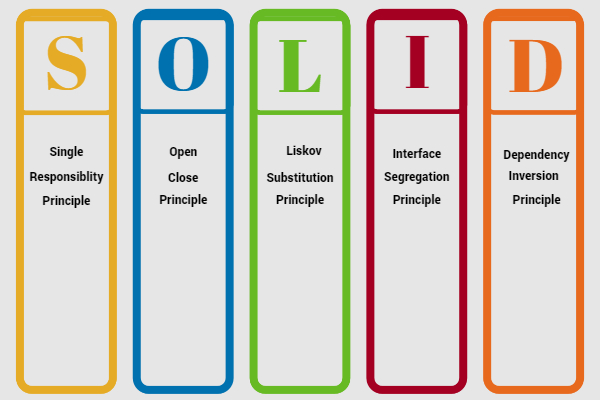Search

# SOLID Principles of OOP: Open-Closed Principle

In this blog, we’ll discuss the Open/Close Principle (OCP) as one of the SOLID principles of object-oriented programming.As the name suggests, this principle states that software entities should be open for extension, but closed for modification. As a result, when the business requirements change then the entity can be extended, but not modified.

For the illustration below, we’ll focus on how interfaces are one way to follow this principle.

For example Let’s consider we’re building a calculator app that might have several operations, such as addition and subtraction. First we will define a interface CalculatorOperationLet’s define an Addition class, which would add two numbers and implement the CalculatorOperation:We define another class named Subtraction:Let’s now define the main class, which will perform the calculator operations.Although the above codes seem fine, this is not a good example of OCP. Because if we add additional classes for multiply and divide , we have no option other than changing the calculate method of the Calculator class.

Hence, we can say this code is not OCP compliant. The code in the calculate method will change with every incoming new operation request. So, we need to extract this code and put it in an abstraction layer.

One solution is to delegate each operation into their respective class: We create an abstract class Perform inside the interface.As a result, the Addition class could implement the logic of adding two numbers by using @Override annotation:Likewise, an updated Subtraction class would have similar logic. And similarly to Addition and Subtraction, we could implement the division logic:And finally, our Calculator class doesn’t need to implement new logic as we introduce new operators:That way the class is closed for modification but open for an extension.